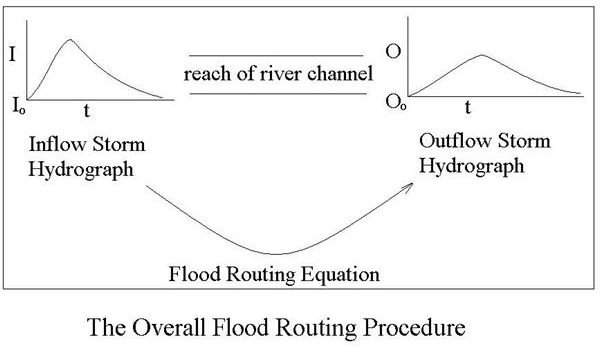# Use of Flood Routing to Predict Downstream Peak River Level using Upstream and Downstream Storm Hydrographs

## Introduction

When a major storm or set of storms take place in the upper part of a river basin, the effects of the storm(s) on the river level in the upper watershed portion of the river become known well before the effects of the storm(s) reach downstream portions of the river basin. For example, if there is a major set of rainstorms in Minnesota, the effects of the level of the Mississippi River in St. Paul become known early on. Flood routing allows prediction of the peak discharge, the peak river level, and the time to peak at downstream points on the river such as Rock Island, St. Louis, and even New Orleans. This article will provide a general description of flood routing procedures using upstream and downstream hydrographs. Some details about the Convex Method flood routing equations will be given.

## Generalized Flood Routing ProcedureThe known upstream information for flood routing should be a storm hydrograph at an upstream location in the river basin, for the storm of interest. A downstream storm hydrograph at a particular point on the river can be generated using the known upstream storm hydrograph together with a flood routing equation, that is an equation relating outflow (O) from the river channel reach between the two points to inflow (I) to that reach, at any time following the beginning of the storm. The equation relating O and I comes from the continuity equation together with information about river channel characteristics (stage-storage and stage-discharge relationships) for the reach of river channel between the upstream and downstream points of interest. Several different flood routing procedures make slightly different assumptions leading to slightly different flood routing equations. The diagram at the left shows the overall flood routing procedure. Once the outflow storm hydrograph is determined, it provides the peak river level and the time to peak.

## Reservoir Routing and Channel Routing

The term flood routing can refer to either reservoir routing or channel routing. Reservoir routing, as the term implies, refers to the process described above with the inlet and outlet hydrographs being at the inlet and outlet to a reservoir or lake. Channel routing, which is described in this article, has a reach of river channel between the inlet and the outlet hydrographs.

## The General Flood Routing Equation

The continuity equation (conservation of mass) states that inflow rate – outflow rate = rate of change of storage, for a fluid system with one or more inflow and outflow streams. Applied to the reach of river channel between the inflow and desired outflow hydrographs, the continuity equation becomes:

½(I1 + I2) – ½(O1 + O2) = (S2 – S1)/Δt …………………………….. (1)

Combining the stage-storage relationships and the stage-discharge relationships at the inlet to and outlet from the reach of channel, leads to the following equation:

S = XKIm/d + (1 – X)KOm/d ………………………………………….. (2)

Where: X, K, m & d are stage-discharge, stage-storage, and channel parameter constants.

## The Convex Method Flood Routing Equation

The convex method uses X = 0 and and m/d = 1 in equation (2) above. Equation (2) becomes: S = KO. Substituting into Equation (1) gives the following Convex Method flood routing equation:

O2 = I1(Δt/K) + O1(1 – Δt/K) ………………………………………… (3)

This equation is usually written as:

O2 = C I1 + (1 – C) O1 ……………………………………………….. (4)

Where C, the convex routing equation constant, is a constant for a given reach of river channel. The channel can be calibrated (value of C determined) using data for both the inflow and outflow hydrographs for one or more storms.

## Determination of an Outflow Hydrograph Using the Convex Method

With a known inflow hydrograph (I vs t), the C value for the channel reach, and an initial value for the outflow hydrograph (O1), the outflow hydrograph can be calculated in a stepwise manner using equation (4).

## About the Author

Dr. Harlan Bengtson is a registered professional engineer with 30 years of university teaching experience in engineering science and civil engineering. He holds a PhD in Chemical Engineering.

## This post is part of the series: Hydrology (The Study of Water)

Hydrology is the study of water. It includes a variety of topics on management of water resources. These include an understanding of the meaning of the term ‘100 year flood’, the steps of the water cylce, a water cycle diagram, and how to predict when a flood crest will reach Town X.